Courses

# Test Level 2: Solid State- 2

## 15 Questions MCQ Test Chemistry Class 12 | Test Level 2: Solid State- 2

Description
This mock test of Test Level 2: Solid State- 2 for Class 12 helps you for every Class 12 entrance exam. This contains 15 Multiple Choice Questions for Class 12 Test Level 2: Solid State- 2 (mcq) to study with solutions a complete question bank. The solved questions answers in this Test Level 2: Solid State- 2 quiz give you a good mix of easy questions and tough questions. Class 12 students definitely take this Test Level 2: Solid State- 2 exercise for a better result in the exam. You can find other Test Level 2: Solid State- 2 extra questions, long questions & short questions for Class 12 on EduRev as well by searching above.
QUESTION: 1

Solution:
QUESTION: 2

### A crystal is made of particle X, Y & Z. X forms FCC packing, Y occupies all octahedral voids of X and Z occupies all tetrahedral voids of X, if all the particles along one body diagonal are removed then the formula of the crystal would be –

Solution:

(D)
When all particle along are body diagonal one removed, these 2 X atoms from corner are removed, one Y particle removed & 2 Z particle removed.

Hence new arrangement, X particle =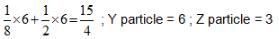Hence formula =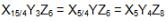QUESTION: 3

### In a hypothetical solid C atoms are found to form cubical close packed lattice. A atoms occupy all tetrahedral vioids & B atoms occupy all octahedral voids. A and B atoms are of appropriate size, so that there is no distortion in CCP lattice of C atoms. Now if a plane as shown in the following figure is cut.Then the cross section of this plane will look like.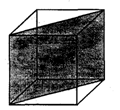Solution:

From the sizes of octahedral and tetrahedral voids, it is clear that the atoms occupying these voids will not touch each other as we have along body diagonal of FCC.

QUESTION: 4

In hexagonal close packing of sphere in three dimensions.

Solution:

(B)
HCP = AB AB AB . pattem repeat For calculating voids between two layers A and B. Total tetrahedral voids = 12 (represented by dots) out of which 8 are completely inside but rest are shared by other unit cells. Total octahedral voids = 6 (represented by cross). All are completely inside.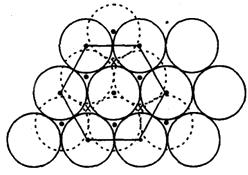QUESTION: 5

Diamond has face-centred cubic lattice. There are two atoms at (0,0,0) and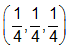coordinates.The ratio of the carbon-carbon bond distance to the edge of the unit cell is

Solution: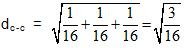and aFCC = 1

QUESTION: 6

The following diagram shows arrangement of lattice point with a = b = c  and α = β = γ  = 900.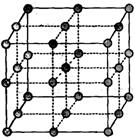Choose the correct options.

Solution:

The correct answer is option A

In a simple cubic structure, the spheres are not packed as closely as they could be, and they only “fill” about 52% of the volume of the container. This is a relatively inefficient arrangement, and only one metal (polonium, Po) crystallizes in a simple cubic structure. A solid with this type of arrangement consists of planes (or layers) in which each atom contacts only the four nearest neighbors in its layer; one atom directly above it in the layer above; and one atom
directly below it in the layer below. The number of other particles that each particle in crystalline solid contact is known as its coordination number. For a polonium atom in a simple cubic array, the coordination number is, therefore, six.

QUESTION: 7

Consider a cube containing n unit cells of a cubic system. A plane ABCD obtained by joining the mid points of the edges on one of its identical faces had atoms arranged as shown. Let p be the packing fraction. Choose the correct option: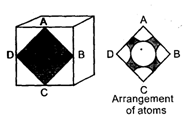Solution:

Cube which contains 8 unit cell.
By observing face ABCP ,we can say that the face of the cube look like in given figure by this P Q R S can be considered as a face of a unit cell which is a simple cubic arrangement.
So n = 8 for this cube.
Since only one atom is present in simple cubic.
In simple cubic 2r = a.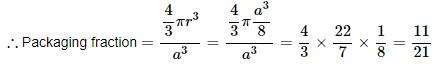QUESTION: 8

Spinel is an important class of oxides consisting of two types of metal ions with the oxide ions arranged in CCP pattern. The normal spinel has 1/ 8 of the tetrahedral holes occupied by one type of metal ion and 1/2 of the octahedral holes occupied by another type of metal ion. Such a spinel is formed by Zn2+, A13x and 02- with Zn2+ in the tetrahedral holes. If CCP arrangement of oxide ions remains undistorted in the presence of all the cations, formula of spinel and fraction of the packing fraction of crystal are respectively :

Solution:

Formula  ZnAl2O4
Packing fraction =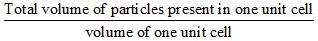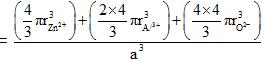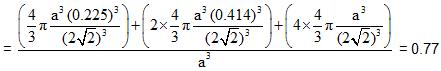QUESTION: 9

What is the maximum number of layers of atoms in close packed planes that will lie within two imaginary parallel planes having a distance between them of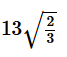R (where R is the radius of atom) in the copper crystal (FCC) ? Consider the atoms to be within the parallel planes if their centres are on or within the two parallel planes.

Solution:

Parallel layer are at a distance ofR

QUESTION: 10

A transition Metal M can exist in two oxidation states +2 and +3.It forms an oxide whose experimental formula is given by MxO where x < 1. Then the ratio of metal ions in +3 state to those in +2 state in oxide is given by :

Solution:

In 1 mole of MxO,
mole of M2+ ion = y
∴ mole of M3+ ion = x - y.
Applying charge balance, 2y + 3(x-y)-2 = 0
⇒ y = 3x - 2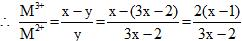QUESTION: 11

Analysis show that nickel oxide consist of nickel ion with 96% ions having d8 configuration and 4% having d7 configuration. Which amongst the following best represents the formula of the oxide.

Solution: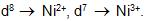Total charge of nickel.
(0.96 x 2) + (0.04 x 3) = 2.04.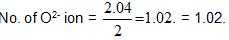Formula of solid = NiO1.02 = Ni0.98O.

QUESTION: 12

PCI5 molecule has

Solution:

Based on theory.

QUESTION: 13

Which of the following statements is correct about the conduction of electricity in pure crystal of silicon at room temperature?

Solution:

At room temperature, thermal energy causes some electrons of the Si-Si bonds to leave.The electron deficient bond becomes a positive hole. Both the positive holes and electrons released account for the conduction of electricity.

QUESTION: 14

Statement-1 : An important feature of Fluorite structures is that cations being large in size occupy FCC lattice points whereas anions occupy all the tetrahedral voids giving the formula unit AB2 (A : cation, B : anion).
Statement-2 : There are 6 cations and 12 anions per FCC unit cell of the Fluorite structure.

Solution:

Based on Fluorite structure of CaF2

QUESTION: 15

Statement-1 : In NaCI crystal each Na ion is touching 6 CI ions but these CI- ions do not touch each other.
Statement--2 : The radius ratio roe rNa+/rCI- is greater than 0.414, required for exact fitting

Solution:

The correct answer is option  A

NaCl type of crystal lattice is a fcc arrangement in which Cl ions and present at the centre of the lattice and at each corner whereas Sodium and is present at each of it's centre.
The radius ratio b/n Na+ and Cl- ion is 0.414 to 0.731